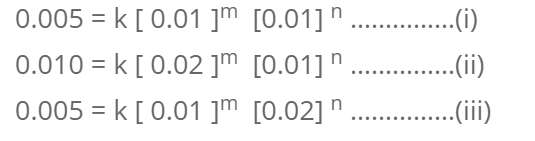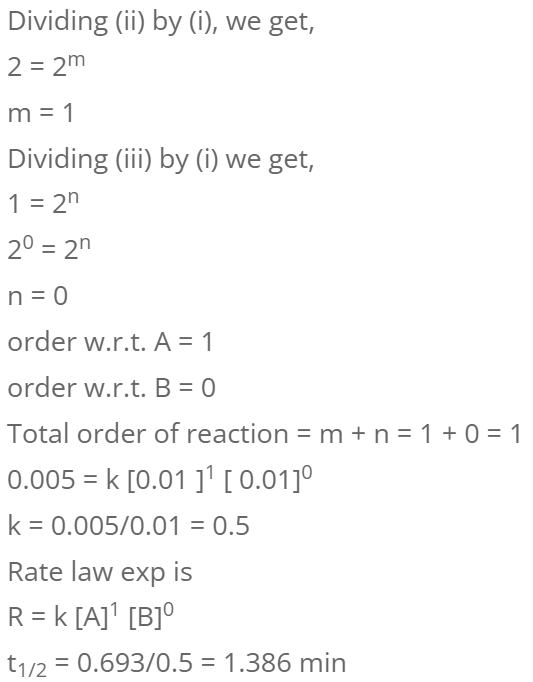# Pseudo Unimolecular Reaction

In a chemical reaction if two or more reactants are involved but the rate of reaction depends only upon the concentration of one of the reactant and independent of other reactants then it is said to be pseudo unimolecular reaction.

Example:

1. Acid Catalyzed Hydrolysis of ester
2. Acid Catalyzed hydrolysis of sucrose

For the reaction, A (g) + B (g) → C(g) + D (g) . It is found that Rate = k [A]2 [B]

How many times does the rate of reaction increase or decrease if

1. The partial pressure of both A and B are double.
2. The partial pressure of A doubles but of B remains constant.
3. The volume of reacting vessel is doubled.
4. An inert gas is added which doubles the overall pressure whilist the partial pressure of A and B remains constant.
5. The temperature rises by 300C.

Soln:

Let the rate of reaction be R. Then,

R = k [A]2 [B]

1. Molar Concentration is directly proportional to partial pressure so if partial pressure of A and B is doubled their concentration is doubled.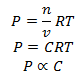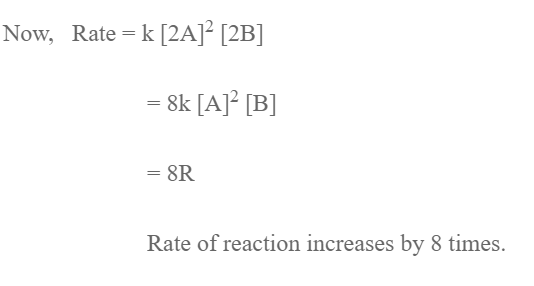2. The concentration of a is doubled while that of B is constant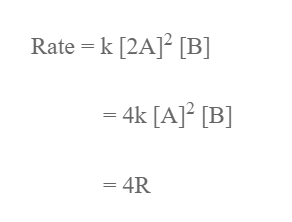Rate of reaction increases by 4 times.

3. When volume of vessel is doubled the concentration of both A and B is halved.

So,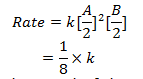Rate of reaction decreases by 8 times.

4. The concentration of A and B remains constant so rate of r ×n is also constant.

5. We know increase in temperature by 10 0c causes increases in rate of reaction by 2-3 times.

For 300C (100C + 100C 100C). The rate increases by 8 (2 ×2×2) to 27 ( 3 ×3×3) times

For the reaction;
A + B → products, following data were obtained.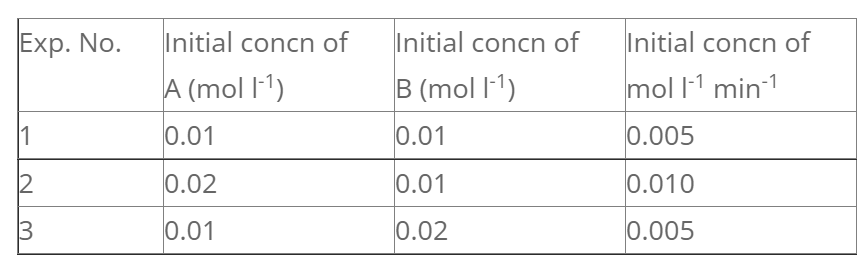Let rate law exp. be,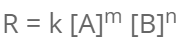We have,